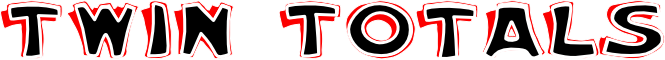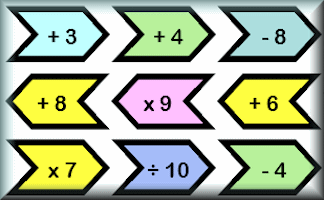#Which two of the following calculations give the same total?

 97 + 1 20 + 71 8 x 3 6 x 8 32 - 14 52 + 58 10 + 101 8 x 1 109 + 59 34 - 13 12 x 11 42 + 77 10 x 12 76 + 73 107 + 97 9 + 35 106 + 52 35 - 15 41 + 3 109 + 48

## A Mathematics Lesson Starter Of The Day

Share

Topics: Starter | Arithmetic

• 7abm2, King Alfred's College, Oxfordshire UK
•
• We enjoyed the starter and we got the correct answer we thought that it was very easy! :).
• Cobham Intermediate, R22 Maths
•
• Too easy, need more of a challenge,
Rate 2.

[Transum: Thanks for your comments. Buttons have been added below to give you the option of larger numbers.]
• Elen Catherine, Ysgol Pen Y Bryn
•
• I love Maths and Starter of the day.

How did you use this starter? Can you suggest how teachers could present or develop this resource? Do you have any comments? It is always useful to receive feedback and helps make this free resource even more useful for Maths teachers anywhere in the world.

If you don't have the time to provide feedback we'd really appreciate it if you could give this page a score! We are constantly improving and adding to these starters so it would be really helpful to know which ones are most useful. Simply click on a button below:

Excellent, I would like to see more like this
Good, achieved the results I required
Satisfactory
Didn't really capture the interest of the students
Not for me! I wouldn't use this type of activity.

This starter has scored a mean of 3.1 out of 5 based on 205 votes.

Previous Day | This starter is for 21 June | Next Day

 97 + 1 = 98 20 + 71 = 91 8 x 3 = 24 6 x 8 = 48 32 - 14 = 18 52 + 58 = 110 10 + 101 = 111 8 x 1 = 8 109 + 59 = 168 34 - 13 = 21 12 x 11 = 132 42 + 77 = 119 10 x 12 = 120 76 + 73 = 149 107 + 97 = 204 9 + 35 = 44 106 + 52 = 158 35 - 15 = 20 41 + 3 = 44 109 + 48 = 157

Note to teacher: Doing this activity once with a class helps students develop strategies. It is only when they do this activity a second time that they will have the opportunity to practise those strategies. That is when the learning is consolidated. Click the button above to regenerate another version of this starter from random numbers.

Your access to the majority of the Transum resources continues to be free but you can help support the continued growth of the website by doing your Amazon shopping using the links on this page. Below is an Amazon search box and some items chosen and recommended by Transum Mathematics to get you started.Teacher, do your students have access to computers?Do they have iPads or Laptops in Lessons? Whether your students each have a TabletPC, a Surface or a Mac, this activity lends itself to eLearning (Engaged Learning).Transum.org/go/?Start=June21

Here is the URL which will take them to a related student activity.

Transum.org/go/?to=chainFor All: